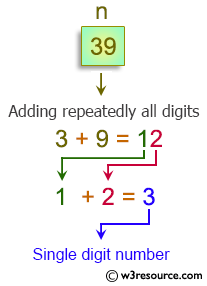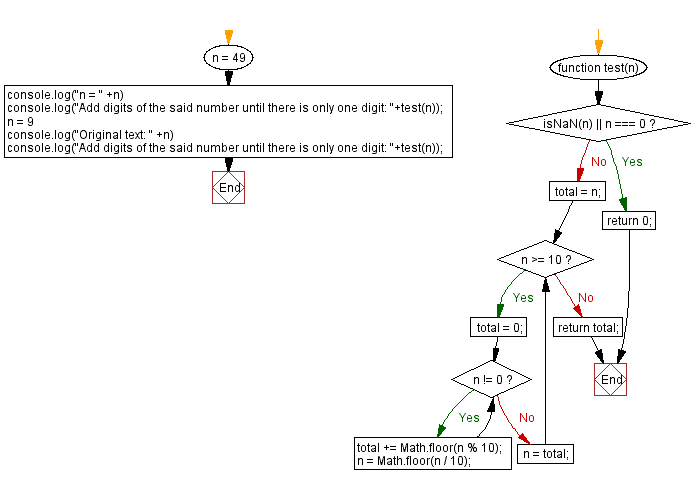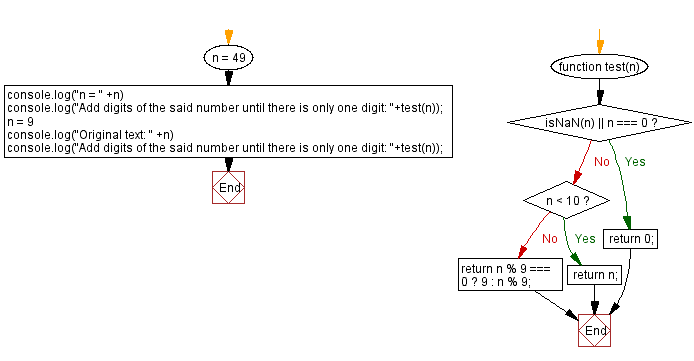# JavaScript: Add digits until there is only one digit

## JavaScript Math: Exercise-99 with Solution

Write a JavaScript program to add repeatedly all the digits of a given non-negative number until the result has only one digit.

For example:
Input: 39
Output: 3
Explanation: The formula is like: 3 + 9 = 12, 1 + 2 = 3.

Visualisation:Test Data:
(47) -> 2
(9) -> 9

Sample Solution-1:

HTML Code:

``````<!DOCTYPE html>
<html>
<meta charset="utf-8">
<title>JavaScript program to Add digits until there is only one digit</title>
<body>

</body>
</html>
```
```

JavaScript Code:

``````function test(n) {
if (isNaN(n) || n === 0) return 0;
total = n;
while (n >= 10) {
total = 0;
while (n != 0) {
total += Math.floor(n % 10);
n = Math.floor(n / 10);
}
n = total;
}

};
n = 49
console.log("n = " +n)
console.log("Add digits of the said number until there is only one digit: "+test(n));
n = 9
console.log("Original text: " +n)
console.log("Add digits of the said number until there is only one digit: "+test(n));
```
```

Sample Output:

```n = 49
Add digits of the said number until there is only one digit: 4
Original text: 9
Add digits of the said number until there is only one digit: 9
```

Flowchart:Live Demo:

See the Pen javascript-math-exercise-98-1 by w3resource (@w3resource) on CodePen.

Sample Solution-2:

JavaScript Code:

``````function test(n) {
if (isNaN(n) || n === 0) return 0;
if (n < 10) return n;
return n % 9 === 0 ? 9 : n % 9;
};
n = 49
console.log("n = " +n)
console.log("Add digits of the said number until there is only one digit: "+test(n));
n = 9
console.log("Original text: " +n)
console.log("Add digits of the said number until there is only one digit: "+test(n));
```
```

Sample Output:

```n = 49
Add digits of the said number until there is only one digit: 4
Original text: 9
Add digits of the said number until there is only one digit: 9
```

Flowchart:Live Demo:

See the Pen javascript-math-exercise-99-1 by w3resource (@w3resource) on CodePen.

Improve this sample solution and post your code through Disqus

What is the difficulty level of this exercise?

Test your Programming skills with w3resource's quiz.

﻿